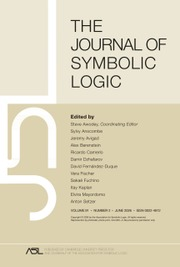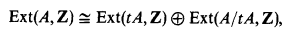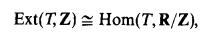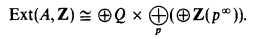Home
Hostname: page-component-5c569c448b-9hjnw Total loading time: 0.262 Render date: 2022-07-06T11:38:41.968Z Has data issue: true Feature Flags: { "shouldUseShareProductTool": true, "shouldUseHypothesis": true, "isUnsiloEnabled": true, "useRatesEcommerce": false, "useNewApi": true } hasContentIssue trueThe Journal of Symbolic Logic

# On the structure of Ext(A,Z) in ZFC+

Published online by Cambridge University Press:  12 March 2014

## Extract

A fundamental problem in the theory of abelian groups is to determine the structure of Ext(A, Z) for arbitrary abelian groups A. This problem was raised by L. Fuchs in 1958, and since then has been the center of considerable activity and progress.

We briefly summarize the present state of this problem. It is a well-known fact thatwhere tA denotes the torsion subgroup of A. Thus the structure problem for Ext(A, Z) breakdown to the two distinct cases, torsion and torsion free groups. For a torsion group T,which is compact and reduced, and its structure is known explicitly .

For torsion free A, Ext(A, Z) is divisible; hence it has a unique representationThus Ext(A, Z) is characterized by countably many cardinal numbers, which we denote as follows: ν0(A) is the rank of the torsion free part of Ext(A, Z), and νp(A) are the ranks of the p-primary parts of Ext(A, Z), Extp(A, Z).

If A is free it is an elementary fact that Ext(A, Z) = 0. The second named author has shown  that in the presence of V = L the converse is also true. For countable torsion free, nonfree A, C. Jensen  has shown that νp(A) is either finite orand νp(A) ≤ ν0(A). Therefore, the case for uncountable, nonfree, torsion free groups A remains to be studied.

Type
Research Article
Information
The Journal of Symbolic Logic , June 1985 , pp. 302 - 315

## Access options

Get access to the full version of this content by using one of the access options below. (Log in options will check for institutional or personal access. Content may require purchase if you do not have access.)

## References

Chase, S., Function topologies on abelian groups, Illinois Journal of Mathematics, vol. 7 (1963), pp. 593608.Google Scholar
Eklof, P., Whitehead's problem is undecidable, American Mathematical Monthly, vol. 83 (1976), pp. 775788.Google Scholar
Eklof, P., Methods of logic in abelian group theory, Abelian group theory (Proceedings, Las Cruces, 1976), Lecture Notes in Mathematics, vol. 616, Springer-Verlag, Berlin, 1977, pp. 251269.Google Scholar
Eklof, P. and Huber, M., Abelian group extensions and the axiom of constructibility, Commentarii Mathematici Hehetici, vol. 54 (1979), pp. 440457.Google Scholar
Eklof, P., On the p-ranks of Ext(A,G), assuming CH, Abelian group theory (Proceedings, Oberwolfach, 1981), Lecture Notes in Mathematics, vol. 874, Springer-Verlag, Berlin, 1981, pp. 93108.Google Scholar
Fuchs, L., Infinite abelian groups. Vol. I, Academic Press, New York, 1970.Google Scholar
Fuchs, L., Infinite abelian groups. Vol. II, Academic Press, New York, 1973.Google Scholar
Hiller, H., Huber, M. and Shelah, S., The structure of Uxt(A, Z) and V = L, Mathematische Zeitschrift, vol. 162 (1978), pp. 3950.Google Scholar
Hiller, H. and Shelah, S., Singular cohomology in L, Israel Journal of Mathematics, vol. 28 (1977), pp. 313319.Google Scholar
Huber, M., Caractérisation des groupes abéliens libres, et cardinalités, Comptes Rendus Hebdomadaires des Séances de l'Académie des Sciences, Séries A et B, vol. 285 (1977), pp. A1A2.Google Scholar
Hulanicki, A., Algebraic characterization of abelian divisible groups which admit compact topologies, Fundamenta Mat hernaticae, vol. 44 (1957), pp. 192197.Google Scholar
Hulanicki, A., Algebraic structure of compact abelian groups, Bulletin de l'Académie Polonaise des Sciences, Séries des Sciences Mathématiques, Astronomiques et Physiques, vol. 6 (1958), pp. 7173.Google Scholar
Jensen, C., Les foncteurs dérivés de lim leurs applications en théorie des modules, Lecture Notes in Mathematics, vol. 254, Springer-Verlag, Berlin, 1972.Google Scholar
Sageev, G. and Shelah, S., On the structure of Ext(A,Z) in L, manuscript, 1980.Google Scholar
Sageev, G., Weak compactness and the structure of Ext(G, Z) in L, Abelian group theory ( Proceedings, Oberwolf ach, 1981), Lecture Notes in Mathematics, vol. 874, Springer-Verlag, Berlin, 1981, pp. 8792.Google Scholar
Shelah, S., Infinite abelian groups—Whitehead problem and some constructions, Israel Journal of Mathematics, vol. 18 (1974), pp. 243256.Google Scholar
Shelah, S., Whitehead groups may not be free, even assuming CH. I, Israel Journal of Mathematics, vol. 28 (1977), pp. 193204.Google Scholar
Shelah, S., On the structure of Ext(G,Z) assuming V = L, manuscript, 1978.Google Scholar
Shelah, S., Whitehead groups may not be free, even assuming CH. II, Israel Journal of Mathematics, vol. 35 (1980), pp. 257285.Google Scholar
Shelah, S., On uncountable abelian groups, Israel Journal of Mathematics, vol. 32 (1979), pp. 311330.Google Scholar
Shelah, S., Consistency of Ext(G,Z) = Q, Israel Journal of Mathematics, vol. 39 (1981), pp. 7482.Google Scholar
27
Cited by

# Save article to Kindle

Note you can select to save to either the @free.kindle.com or @kindle.com variations. ‘@free.kindle.com’ emails are free but can only be saved to your device when it is connected to wi-fi. ‘@kindle.com’ emails can be delivered even when you are not connected to wi-fi, but note that service fees apply.

Find out more about the Kindle Personal Document Service.

On the structure of Ext(A,Z) in ZFC+
Available formats
×

# Save article to Dropbox

To save this article to your Dropbox account, please select one or more formats and confirm that you agree to abide by our usage policies. If this is the first time you used this feature, you will be asked to authorise Cambridge Core to connect with your Dropbox account. Find out more about saving content to Dropbox.

On the structure of Ext(A,Z) in ZFC+
Available formats
×

# Save article to Google Drive

To save this article to your Google Drive account, please select one or more formats and confirm that you agree to abide by our usage policies. If this is the first time you used this feature, you will be asked to authorise Cambridge Core to connect with your Google Drive account. Find out more about saving content to Google Drive.

On the structure of Ext(A,Z) in ZFC+
Available formats
×
×